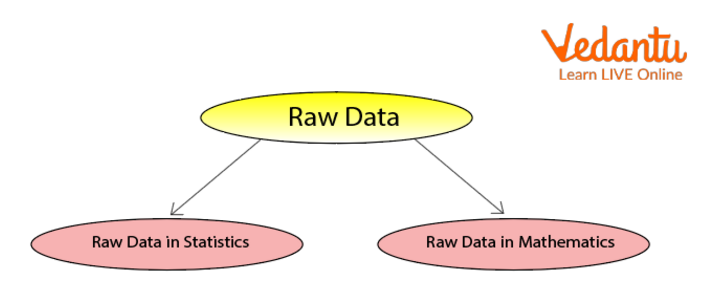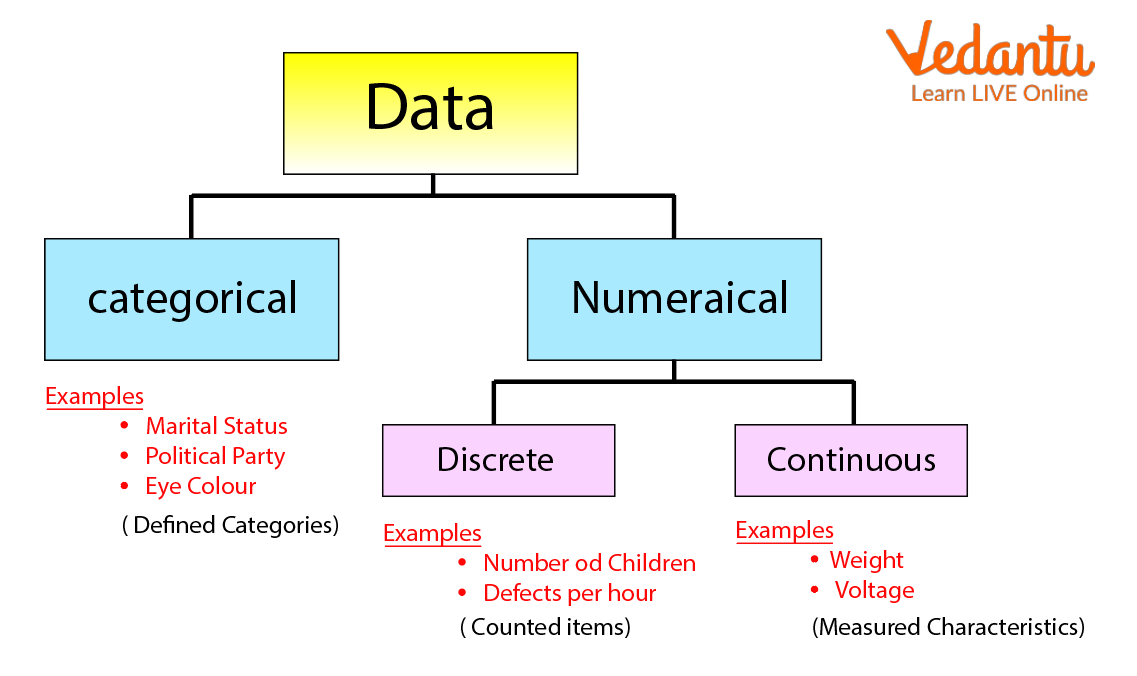Courses
Courses for Kids
Free study material
Free LIVE classes
More

# Raw Data in StatisticsLIVE
Join Vedantu’s FREE Mastercalss

## Definition of Data

Data is a sort of information that is used to represent the figures, such as facts and numbers to analyse something and to take decisions on behalf. The data can be read in two terms: raw data in statistics and raw data in mathematics.Raw Data

## Raw Data in Statistics

In statistics, raw data can be defined as the data that has been collected directly from a primary source of data that is not processed or cleaned by anyone. The first step is to collect the data from the primary source of data and then when the data is gathered, it can be cleaned and summarised in various forms.

## Terms Related to Statistics

Data: The collection of information in the form of numbers is known as data.

Observation: All the collection of numerical facts in given data is known as observation.

Array: Putting the raw data in ascending or descending order is known as an array. Example: \[4,8,1,6,5,4\;\;\;\] Array: Keeping the above data in ascending order: \[1,4,4,5,6,8\].

Range: Range is the difference between the highest number and the lowest number. For example, the highest number is 45 and the lowest number is 15. Hence, the range is \[45 - 15 = 30.\]

## Raw Data Examples in Statistics

• The grades of all the students in a class.

• List of music being streamed on the radio.

• Amount collected by students for teachers’ day function.

These are the common examples of raw data in statistics. Generally, all the data is used to make a better understanding and make decisions upon it.

## Raw Data in Mathematics

The data was collected in an unorganised form. if the data is collected in numerical value, it is quantitative raw data and the data collected by non-numerical values is called qualitative raw data. The given diagram will give you a slightly better understanding about the data and its types in Mathematics.Data

## Raw Data Examples in Mathematics

• The marks obtained by 5 students in Maths tests: \[45,23,56,45,78\].

• The number of apples in one kilogram of apples.

• The number of bananas in one dozen bananas.

These all examples can be measured in numerical form.

## Solved Questions

1. What is Raw Data?

Raw data is data that has been collected by a primary source. It is the first data collected by using a primary source; it is also known as primary data.

2. Name two terms related to statistics.

The two terms related to statistics are observation and array.

3. What is qualitative data?

The data collected in numerical value means it is quantitative raw data.

4. Give one example of raw data?

The number of apples in one kilogram of apples.

## Summary

The raw data is the unorganised data that is collected by the primary source of data. The raw data can be qualitative and quantitative. The raw data can be studied in Statistics or Mathematics. There are many terms in statistics related to raw data. For example, Data, Array, Range etc.

Last updated date: 26th Sep 2023
Total views: 94.2k
Views today: 0.94k

## FAQs on Raw Data in Statistics

1. What is Array?

Putting the raw data in ascending or descending order is known as an array.

2. Can raw data be qualitative?

Yes, raw data can be quantitative and quantitative.

3. Raw data is in an organised form. True or false?

False, raw data is in an unorganised form.